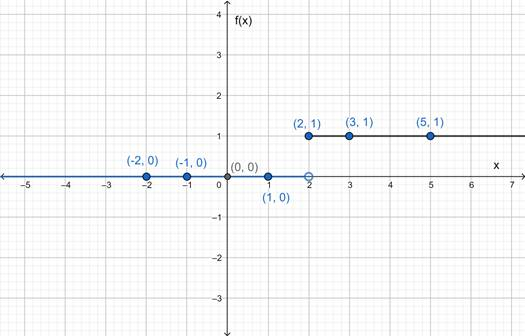# The graph of the given function.### Precalculus: Mathematics for Calcu...

6th Edition
Stewart + 5 others
Publisher: Cengage Learning
ISBN: 9780840068071### Precalculus: Mathematics for Calcu...

6th Edition
Stewart + 5 others
Publisher: Cengage Learning
ISBN: 9780840068071

#### Solutions

Chapter 2.2, Problem 33E
To determine

## To sketch : The graph of the given function.

Expert Solution

### Explanation of Solution

Given information :

f(x)={0  if  x<21  if  x2

Graph :

 x 0 1 2 3 5 −1 −2 f(x) 0 0 1 1 1 0 0The above curve is the graph of the given equation.

Interpretation : f(x) has two values. When the value of x is less than 2 , the value of f(x) is 0 (zero). Otherwise the value of f(x) is 1 .

### Have a homework question?

Subscribe to bartleby learn! Ask subject matter experts 30 homework questions each month. Plus, you’ll have access to millions of step-by-step textbook answers!